# AP Statistics : How to define a Type I error

## Example Questions

### Example Question #5 : Defining Errors

A prominent football coach is being reviewed for his performance in the past season. To evaluate how well the coach has done, the team manager runs a statistical test comparing the coach to a sample of coaches in the league. If the test suggests that the coach outperformed other coaches when in fact he did not, and the manager then rejects the null hypothesis (that the coach did not outperform the other coaches), what kind of error is he committing?

Type I error

Type M error

None of the above

Type II error

Type I error

Explanation:

A type I error occurs when one rejects a null hypothesis that is in fact true. The null hypothesis is that the coach does not outperform other coaches, and the test reccomends that we reject it even though it is true. Thus, a type I error has been committed.

### Example Question #6 : Defining Errors

If a hypothesis test uses a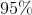confidence level, then what is its probability of Type I Error?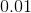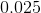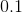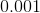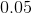Explanation:

By definition, the probability of Type I Error is,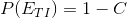where,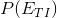represents Probability of Type I Error andrepresents the confidence level.

Thus resulting in: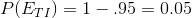### Example Question #7 : Defining Errors

For significance tests, which of the following is an incorrect way to increase power (the probability of correctly rejecting the null hypothesis)?

Increase sample size

Decrease the probability of making a Type I error

Increase the probability of making a Type I error

Increase the difference between the null hypothesis and the alternative hypothesis

Decrease variability of samples

Decrease the probability of making a Type I error

Explanation:

Recall that power is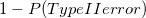. The probability of Type I and Type II errors will change inversely of each other as the probability of making a Type I error changes. If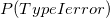increases, then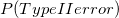decreases, and as a result power will increase. So ifdecreases,would increase, and power would decrease; therefore decreasingwill not increase power.

### Example Question #8 : Defining Errors

In a recent athletic study, your lab partner told you that they rejected the null hypothesis that the fabric of running shoes has no effect on the wearer's running times.

If the null hypothesis was actually valid, what type of error was made?

Type I and II

Type II

Neither

Type I

Type I

Explanation:

A type I error occurs when the null hypothesis is valid but rejected.

A type II error occurs when the null hypothesis is false, but fails to be rejected.

Because the null hypothesis was true, but rejected, they made a Type I error.

### Example Question #9 : Defining Errors

In a recent academic study, your lab partner told you that they rejected the null hypothesis that the ionization of water has no effect on the rate of grass growth.

If the null hypothesis was actually valid, what type of error was made?

Neither

Type II

Type I and II

Type I

Type I

Explanation:

A type I error occurs when the null hypothesis is valid but rejected.

A type II error occurs when the null hypothesis is false, but fails to be rejected.

Because the null hypothesis was true, but rejected, they made a Type I error.

### Example Question #10 : Defining Errors

A company claims that they have 12 ounces of potato chips in each of their bags of chips.  A customer complaint is filed that they do not truly contain 12 ounces but actually contain less.  A sampling test is conducted to see if the comapny measure is true or not. What would be an example of a Type I error?

The test finds there are less than 12 ounces but there are actually 12 ounces.

There test does not come up with a defined answer.

The test finds that there are 12 ounces in each but the true amount is less than that.

The test finds that there is less than 12 ounces and the customer claim was indeed true.

There are 12 ounces in each bag and the test backs the company claim.